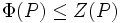# Frattini-in-center odd-order p-group implies (mp plus 1)-power map is automorphism

This article states and (possibly) proves a fact that is true for odd-order p-groups: groups of prime power order where the underlying prime is odd. The statement is false, in general, for groups whose order is a power of two.
View other such facts for p-groups|View other such facts for finite groups

## Statement

Suppose$p$ is an odd prime, and$P$ is a finite$p$-group (i.e., an Odd-order p-group (?): a Group of prime power order (?) with an odd prime). Then, if$P$ is a Frattini-in-center group (?), i.e., if$\Phi(P) \le Z(P)$, and$m$ is any integer, then the map$g \mapsto g^{mp+1}$ is an automorphism of$P$.

Thus, it is a universal power automorphism. In the particular case that$P$ does not have exponent$p$, this gives a non-identity universal power automorphism.

## Examples

For any odd prime$p$, the smallest non-abelian examples are the groups of order$p^3$. There are two such examples: prime-cube order group:U(3,p) (GAP ID$(p^3,3)$) and semidirect product of cyclic group of prime-square order and cyclic group of prime order (GAP ID$(p^3,4)$). The former has exponent$p$, so the$(p+1)$- power map is the identity automorphism. The latter has exponent$p^2$, so that$(p+1)$-power map is a non-identity universal power automorphism. In fact, this automorphism itself has order$p$.

For the case$p = 3$, these groups become prime-cube order group:U(3,3) and semidirect product of Z9 and Z3 respectively, both of order 27.

## Proof

This proof uses a tabular format for presentation. Provide feedback on tabular proof formats in a survey (opens in new window/tab) | Learn more about tabular proof formats|View all pages on facts with proofs in tabular format

Given: An odd prime$p$, a finite$p$-group$P$ that is a Frattini-in-center group, i.e.,$P/Z(P)$ is elementary abelian, or equivalently,$\Phi(P) \le Z(P)$.$m$ is an integer.

To prove: The map$g \mapsto g^{mp + 1}$ is an automorphism of$P$.

Proof:

Step no. Assertion Facts used Given data used Previous steps used Explanation
1 Define$\tau$ as the map$g \mapsto g^p$. Then$\tau$ is an endomorphism of$P$. Fact (1)$P$ is Frattini-in-center, and$p$ is odd. -- Fact+Given direct.
2 The image of$\tau_0:= g \mapsto g^p$ is in$Z(P)$.$P$ is Frattini-in-center. [SHOW MORE]
3 The map$\tau:g \mapsto g^{mp}$ is an endomorphism of$P$ taking values in$Z(P)$. Fact (2) Steps (1), (2) [SHOW MORE]
4 The map$\sigma:= g \mapsto g^{mp + 1}$ is an endomorphism of$P$. Fact (3) Step (3) Fact+Step direct, setting$n = mp + 1$.
5 The map$\sigma := g \mapsto g^{mp+1}$ is bijective from$P$ to$P$. Fact (4) [SHOW MORE]
6 The map$\sigma := g \mapsto g^{mp+1}$ is an automorphism of$P$. Steps (4), (5) Step-combination-direct.